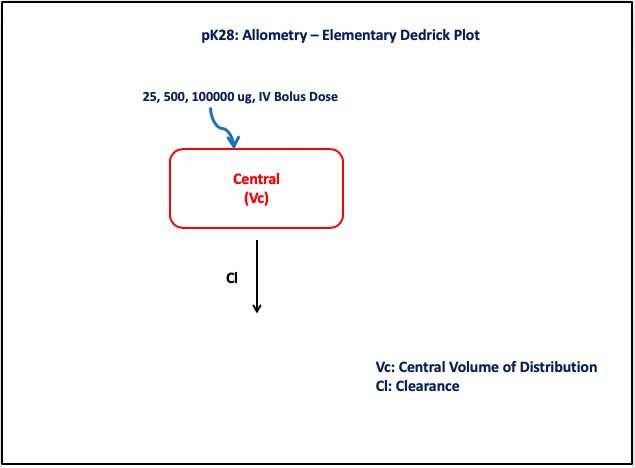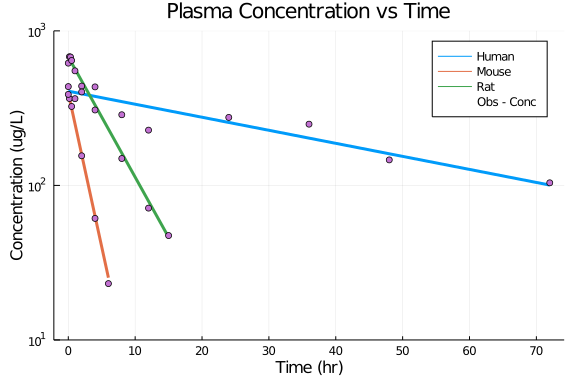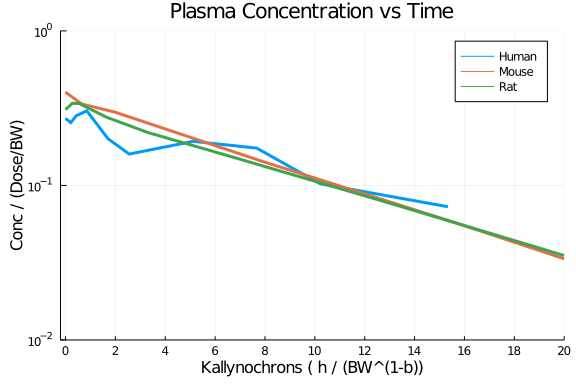# Exercise PK28 - Allometry - Elementary Dedrick Plot

### Background

• Structural model - One Compartment Model with Linear Elimination

• Route of administration - IV-Bolus

• Dosage Regimen - 25, 500, 100000 μg adminstered after complete washout

• Number of Subjects - 3 species (Mouse, Rat, Human)### Learning Outcome

• To learn about Allometry - Elementary Dedrick Plots

• To simulatenously fit an allometric model to concentration-time data obtained from three different species

### Objectives

• To apply the allometric constants a,b,c,d

• To obtain Cl based on the typical values of a,b and Body Weight

• To obtain V based on the typical values of c,d and Body Weight

### Libraries

call the "necessary" libraries to get started

using Pumas
using Plots
using CSV
using StatsPlots
using Random


### Model

The given data follows a One-Compartment Model with linear eliminiation

pk_28       = @model begin
@param begin
a       ∈ RealDomain(lower=0)
b       ∈ RealDomain(lower=0)
c       ∈ RealDomain(lower=0)
d       ∈ RealDomain(lower=0)
Ω       ∈ PDiagDomain(2)
σ²_prop ∈ RealDomain(lower=0)
end

@random begin
η       ~ MvNormal(Ω)
end

@covariates BW

@pre begin
Cl      = a * (BW)^b * exp(η)
Vc      = c * (BW)^d * exp(η)
end

@dynamics begin
Central'= -(Cl/Vc)*Central
end

@derived begin
cp      = @. Central/Vc
dv      ~ @. Normal(cp, sqrt(cp^2*σ²_prop))
nca    := @nca cp
AUC     = NCA.auc(nca)
CL      = NCA.cl(nca)
V       = NCA.vz(nca)
t_half  = NCA.thalf(nca)
end
end

PumasModel
Parameters: a, b, c, d, Ω, σ²_prop
Random effects: η
Covariates: BW
Dynamical variables: Central
Derived: cp, dv, AUC, CL, V, t_half
Observed: cp, dv, AUC, CL, V, t_half


### Parameters

• a - typical value of clearance among the species

• b - scaling parameter for Cl

• c - typical value of volume of distribution among the species

• d - scaling parameter for Vc

param = ( a       = 0.319142230070251,
b       = 0.636711976371785,
c       = 3.07665859278123,
d       = 1.03093780182922,
Ω       = Diagonal([0.0,0.0]),
σ²_prop = 0.01)

(a = 0.319142230070251, b = 0.636711976371785, c = 3.07665859278123, d = 1.
03093780182922, Ω = [0.0 0.0; 0.0 0.0], σ²_prop = 0.01)


### Dosage Regimen

• Species 1 (Mouse) - 25μg IV-Bolus and bodyweight (23grams)

• Species 2 (Rat) - 500μg IV-Bolus and bodyweight (250grams)

• Species 3 (Human) - 100000μg IV-Bolus and bodyweight (70kg)

ev1      = DosageRegimen(25, cmt=1, time=0, route=NCA.IVBolus)
sub1     = Subject(id="Mouse", events=ev1, covariates=(BW=0.023,), time=[0,0.167,0.5,2,4,6])
ev2      = DosageRegimen(500, cmt=1, time=0,route=NCA.IVBolus)
sub2     = Subject(id="Rat", events=ev2, covariates=(BW=0.250,), time=[0,0.167,0.33,0.5,1,2,4,8,12,15])
ev3      = DosageRegimen(100000, cmt=1, time=0, route=NCA.IVBolus)
sub3     = Subject(id="Human", events=ev3, covariates=(BW=70,), time=[0,1,2,4,8,12,24,36,48,72])
pop3_sub = [sub1,sub2,sub3]

Population
Subjects: 3
Covariates: BW


### Simulation

Random.seed!(123)
sim_pop3 = simobs(pk_28, pop3_sub, param)
df1      = DataFrame(sim_pop3)


### Dataframe and Plotting

A plot of Concentration vs Time data from three different species

@df df1 plot(:time, :cp, yaxis=:log, group=:id, linewidth=3,
title="Plasma Concentration vs Time", xlabel="Time (hr)", ylabel="Concentration (ug/L)",
yticks=[10,100,1000], ylims=(10,1000),xticks=[0,10,20,30,40,50,60,70,80])
@df df1 scatter!(:time, :dv, yaxis=:log, label="Obs - Conc" )An Elementary-Dedrick Plot of dose and bodyweight normalized plasma concentration vs bodyweight normalized time

transform!(groupby(df1, :id), :amt => first => :amt)
dropmissing!(df1, :cp)
df1.amt_bw  = df1.amt ./ df1.BW
df1.yfactor = df1.dv ./ df1.amt_bw
df1.bw_b    = df1.BW .^(1-0.636)
df1.xfactor = df1.time ./ df1.bw_b

@df df1 plot(:xfactor, :yfactor, yaxis=:log, group=:id,
xlabel="Kallynochrons ( h / (BW^(1-b))", ylabel="Conc / (Dose/BW)",
title="Plasma Concentration vs Time", linewidth=3,
xticks=[0,2,4,6,8,10,12,14,16,18,20], xlims=(-0.2,20), yticks=[0.01,0.10,1.00], ylims=(0.01,1.00))